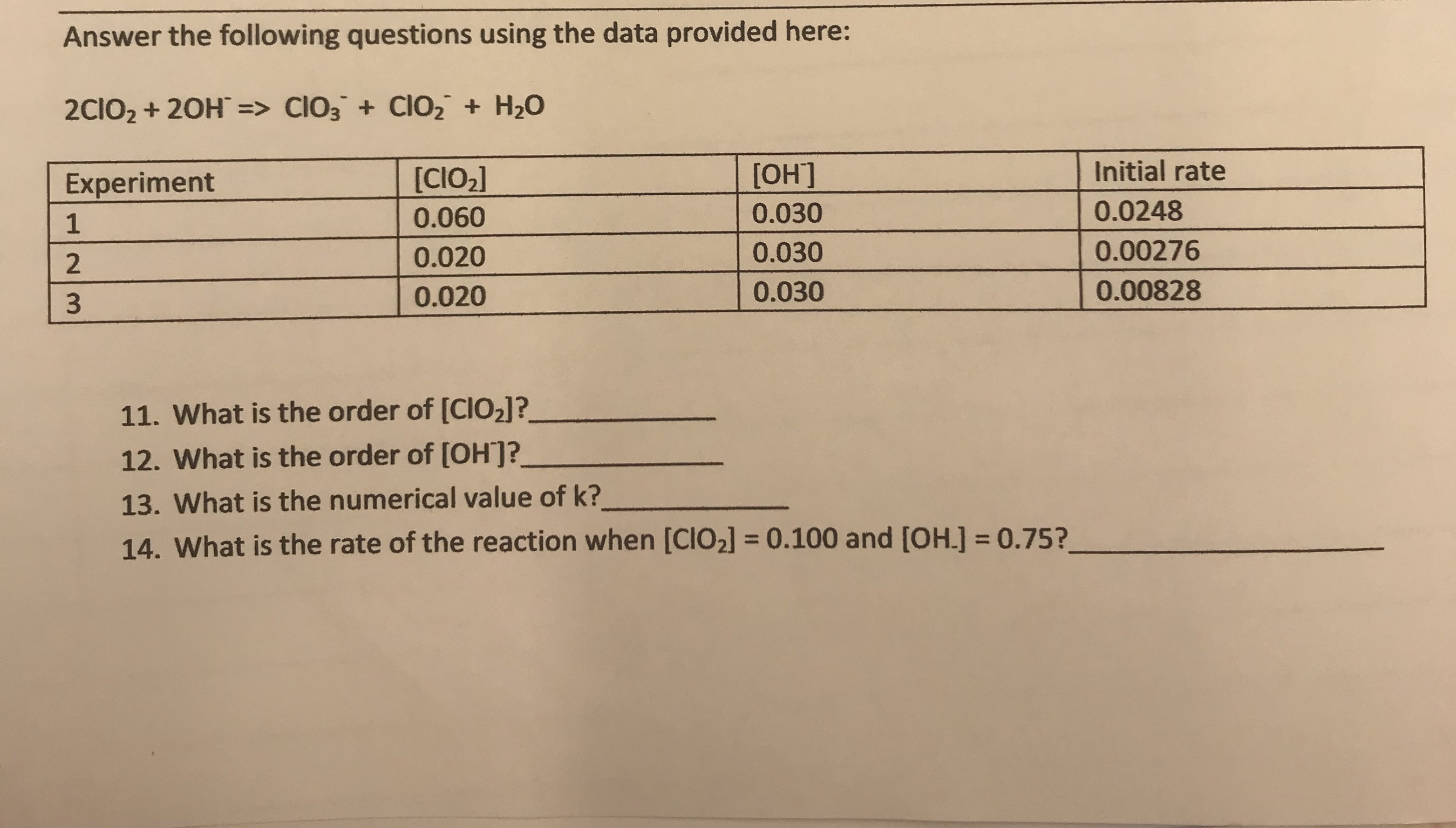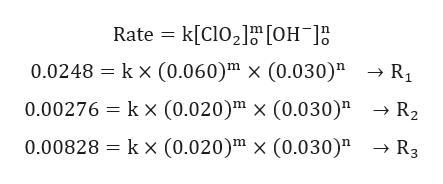Answer the following questions using the data provided here:2CIO2 20H => CIO3 Cl02 H20Initial rate[ОН][CIO2]Experiment0.02480.0300.06010.002760.0300.0200.008280.0300.02011. What is the order of [CIO2]?12. What is the order of [OH?13. What is the numerical value of k?14. What is the rate of the reaction when [CIO2] = 0.100 and [OH.] 0.75?

Question

What is the rate of the reaction when [ClO2] = 0.100 and [OH-] = 0.75?help_outlineImage TranscriptioncloseAnswer the following questions using the data provided here: 2CIO2 20H => CIO3 Cl02 H20 Initial rate [ОН] [CIO2] Experiment 0.0248 0.030 0.060 1 0.00276 0.030 0.020 0.00828 0.030 0.020 11. What is the order of [CIO2]? 12. What is the order of [OH? 13. What is the numerical value of k? 14. What is the rate of the reaction when [CIO2] = 0.100 and [OH.] 0.75? fullscreen
Step 1

Note: please verify the given concentration depending on initial rate of reaction. In the trail-3 the [OH-] should be 0.09 as rate of reaction is increasing by 3 times.

Step 2

Given reaction:

Step 3

Rate Law is gi...help_outlineImage TranscriptioncloseRate k[ClO2 [OH R1 0.0248 kx (0.060)m x (0.030)" 0.00276 kx (0.020)" x (0.030)" R3 0.00828 kx (0.020)" x (0.030)" fullscreen

Want to see the full answer?

See Solution

Want to see this answer and more?

Our solutions are written by experts, many with advanced degrees, and available 24/7

See Solution
Tagged in

Chemical Kinetics